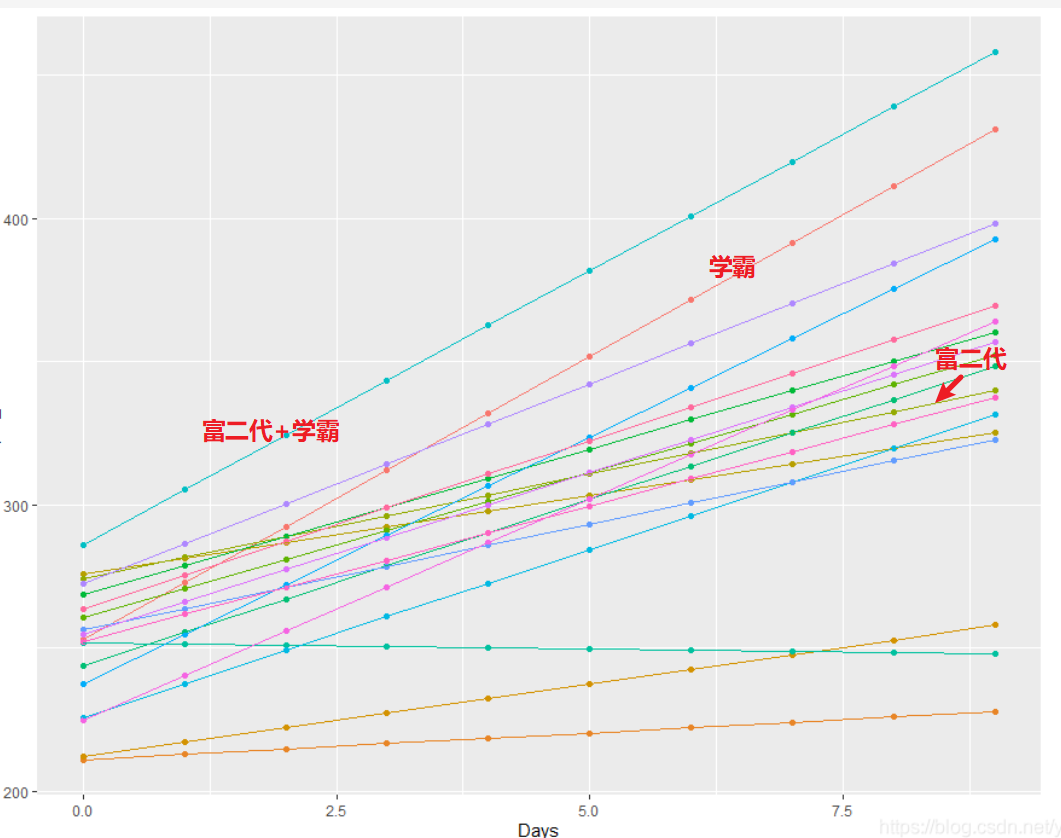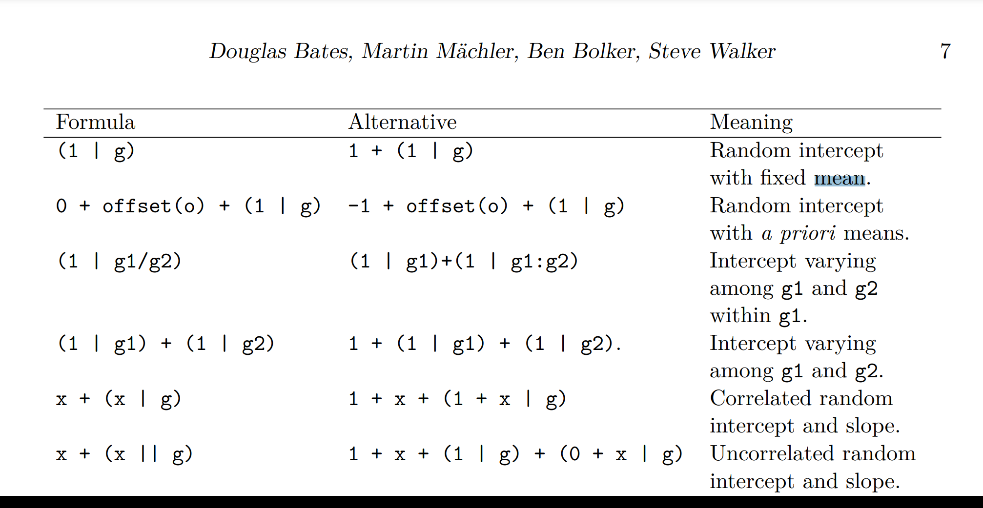# 混合线性模型的理解 | 学霸和富二代的故事

## 富二代和学霸的故事

「学生分类：」

• 富二代，富二代家里有钱，幼儿园和小学都有私人教师，个性化补习，起点较高。
• 学霸，学霸就是聪明，知识掌握容易，进步很快
• 普通学生，起点一般，知识掌握速度也一般

「对应模型：」

lmer混合线性模型中，随机因子的定义有「截距，intercept」「斜率，slope」两个参数。## 1. 模型解释

lmer常用模型公式如下：

mod= lmer(data = , formula = y ~ Fixed_Factor + (Random_intercept + Random_Slope | Random_Factor))
• data，为数据集
• y，为观测值，所要分析的性状，因变量
• Fixed_Factor，为固定因子
• ()内为随机因子
• Random_intercept，为随机截距，即认为不同群体因变量的分布不同（通俗的解释：有的人生下来起点高，是富二代，有的人是一般群众，起点低）
• Random_Slope，为随机斜率，即认为不同群体受固定因子的影响不同（通俗解释：有的人是学霸，学习能力强，2个小时学会，斜率高；有的人是学渣，2天才能学会，斜率低）
• Random_Factor，随机因子

## 2. 常用模型

### 2.1 Random intercept with fixeed mean

• (1 | g)
• 也可以写为：1 + （1 | g）

mod1a = lmer(Reaction ~ Days + (1 | Subject), data=dat)
mod1aa = lmer(Reaction ~ Days + 1 + (1 | Subject), data=dat)

### 2.2 Random intercept with a priori means

• 0 + offset(0) + (1 | g)
• 也可以写为：-1 + offset(0) + (1 | g)

The names of grouping factors are denoted g, g1, and g2, and covariates and a priori known offsets as x and o

### 2.3 Intercept varying among g1 and g2 within g1

• 1 + (1 | g1/g2)
• 也可以写为：(1 | g1) + (1 | g1:g2)

### 2.4 Intercept varying among g1 and g2

• (1 | g1) + (1|g2)
• 也可以写为：1 + (1 | g1) + (1|g2)

### 2.5 Correlated random intercept and slope

• x + (x | g)
• 也可以写为：1 + x + (1 + x|g)

### 2.6 Uncorrelated random intercept and slope

• x + (x || g)
• 也可以写为：1 + x + (1|g) + (0 + x|g)

「公式汇总：」「注意：」这里，x为数值协变量，gg1g2为因子协变量。

https://wap.sciencenet.cn/blog-2577109-1289878.html

## 全部精选博文导读

GMT+8, 2021-7-26 13:50